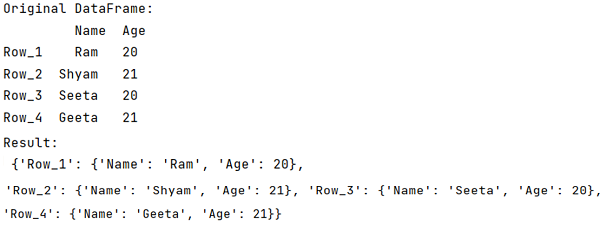# How to convert rows in DataFrame in Python to dictionaries?

Given a Pandas DataFrame, we have to convert its rows to dictionaries.
By Pranit Sharma Last updated : September 24, 2023

Pandas is a special tool that allows us to perform complex manipulations of data effectively and efficiently. Inside pandas, we mostly deal with a dataset in the form of DataFrame. DataFrames are 2-dimensional data structures in pandas. DataFrames consist of rows, columns, and data.

## Problem statement

Given a Pandas DataFrame, we have to convert its rows to dictionaries.

## Solution

We know that pandas.DataFrame.to_dict() method is used to convert DataFrame into dictionaries, but suppose we want to convert rows in DataFrame in python to dictionaries.

Syntax:

```DataFrame.to_dict(orient='dict', into=<class 'dict'>)
```

## Converting rows in DataFrame in Python to dictionaries

To achieve this task, we will first create a DataFrame and then we will use the same method i.e., pandas.DataFrame.to_dict() but this time we will pass a parameter 'orient' = 'index', it will take the rows of DataFrame as the parent key of dictionary and columns as child dictionary along with their values.

Note

To work with pandas, we need to import pandas package first, below is the syntax:

```import pandas as pd
```

Let us understand with the help of an example,

## Python program to convert rows in DataFrame in Python to dictionaries

```# Importing pandas package
import pandas as pd

# Creating a dictionary
d = {
'Name':['Ram','Shyam','Seeta','Geeta'],
'Age':[20,21,20,21]
}

# Creating a DataFrame
df = pd.DataFrame(d,index=['Row_1','Row_2','Row_3','Row_4'])

# Display original DataFrame
print("Original DataFrame:\n",df,"\n")

# Converting rows of DataFrame into dictionaries
result = df.to_dict(orient='index')

# Display result
print("Result:\n",result)
```

## Output

The output of the above program is: